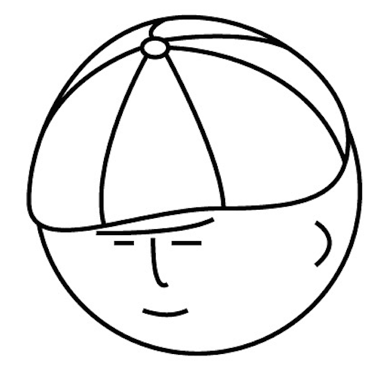マツシタのお勉強メモ

マツシタのお勉強

welcome to my engineering blog
Hacker RankProblem

Imagine a robot sitting on the upper left corner of an X by Y grid. The robot can only move in two directions: right and down. How many possible paths are there for the robot to go from (0, 0) to (X, Y)?

How to Solve

By using Dynamic Programming, we can solve this problem efficiently. First we can make recurrence relation like this.

memo(i, j) = memo(i - 1, j) + memo(i, j -1)

memo(i, j) is defined that the count of paths from coordinate(i, j) to (X, Y).